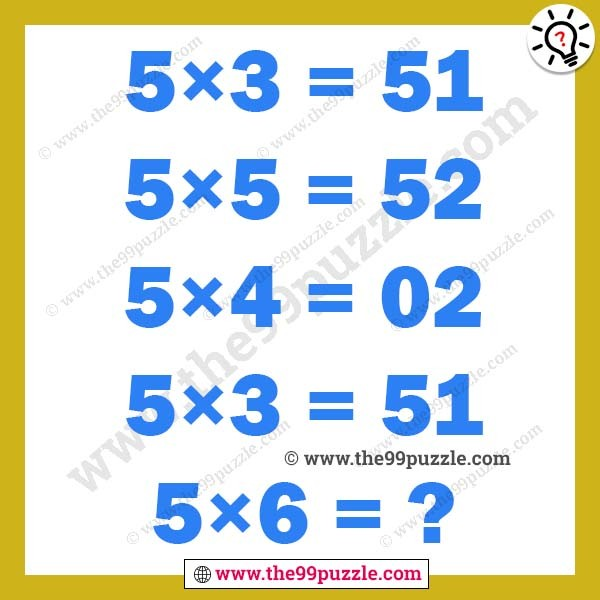# Logical reasoning math puzzles for adults – Puzz147

Can you solve the logical reasoning math puzzles? These types of puzzles can challenge your mind. If you are intelligent you can solve the logical reasoning math puzzles. Find out the value of the last equation?

5×3=51

5×5=52

5×4=02

5×3=51

5×6=?###### Explanation:

5×3=15(Reverse the number)=51

5×5=25(Reverse the number)=52

5×4=20(Reverse the number)=02

5×3=15(Reverse the number)=51

5×6=30(Reverse the number)=03